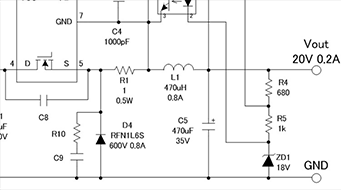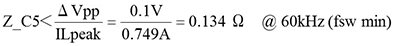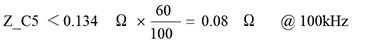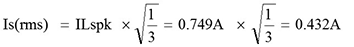Technical Information Site of Power Supply Design

• Basic Knowledge
• AC/DC
• Design Examples of Non-isolated Buck Converters
• Selecting Critical Components: Output Capacitor C5

2018.01.11 AC/DC

# Selecting Critical Components: Output Capacitor C5

Design Examples of Non-isolated Buck Converters

An output capacitor, in conjunction with an inductor, forms an LC filter to smooth the output voltage, and also serves to supply load current. In addition, the magnitude of the output ripple voltage depends heavily on the capacitor impedance.

Output Capacitor C5

The circuit diagram on the right is an excerpt of the output area. The output capacitor C5 is connected between the output and GND. As is obvious, it forms an LC filter together with the inductor.

When the MOSFET within the IC is turned on, the output diode D4 is turned off, the output capacitor is charged, and a load current is supplied.

When the MOSFET is turned off, the output diode is turned on, and at this time the output capacitor supplies the load current.Calculation of the Output Capacitor Value

The output capacitor should be selected such that the peak ripple voltage (ΔVpp) of the output is within the output ripple voltage that is the design target. The output ripple voltage is determined by the ripple current, which is the effective value of the peak inductor current, and the capacitor impedance. Hence calculations begin from the targeted ripple voltage. The impedance Z of C5 can be calculated using the following equation.

if ΔVpp = 100 mV：The value of Z thus determined is the value when the minimum switching frequency of this circuit is 60 kHz. The condition when specifying the impedance of an electrolytic capacitor (low-impedance component) for switching power supplies in general is 100 kHz. The capacitor impedance decreases approximately linearly with frequency up to the resonance point, and so the following equation can be used to determine Z for 100 kHz.Next, we determine the ripple current Is(rms).With this, we have determined the capacitor impedance and the ripple current.

Next, as an empirical rule, the rated voltage is generally set to about twice the output voltage.

VOUT × 2 = 20 V × 2 = 40 V →　35 V or higher

Thus we must select an electrolytic capacitor that satisfies the requirements of an impedance of 0.08 Ω or lower, a rated ripple current of 0.4 A or higher, and a rated voltage of 35 V or above. In this circuit, a 470 μF electrolytic capacitor for switching power supplies with low impedance and a rated voltage of 35 V was selected.

Not limited to output capacitors, it is always important to confirm the actual ripple voltage and ripple current in the actual circuit.

In addition, electrolytic capacitors are components with limited lifetimes, and if they pass large ripple currents, that lifetime is shortened. Where product lifetime is concerned, the capacitor manufacturer should provide calculation methods and rules, and so the manufacturer of the capacitor used should be consulted.

#### Key Points:

・An output capacitor is selected based on the ripple current and capacitor impedance, so as to satisfy the output ripple voltage design target.

・Aluminum electrolytic capacitors are components with limited lifetimes, and the lifetime is shortened by large ripple currents.

This website uses cookies.

By continuing to browse this website without changing your web-browser cookie settings, you are agreeing to our use of cookies.